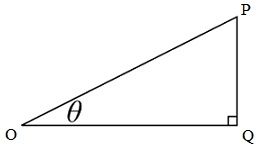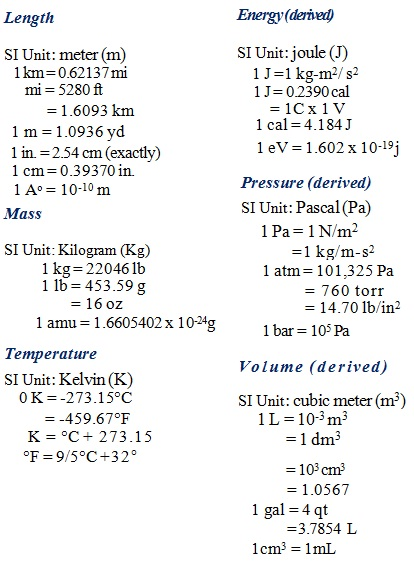#### SI prefixes, Chemistry tutorial

Introduction to SI Prefixes:

In this, we are now going to illustrate how to overcome the difficulty of expressing the units of physical quantities that are either extremely large or small as compared to the SI units. We add a prefix to the unit in such a way that the magnitude of the physical quantity is 7. 4 x 10-12 m. We represent or illustrate it conveniently as 74 pm where pico is the SI prefix and p is its Symbol. The list of SI prefixes is represented in the table shown below and it is possible to change the order of magnitude of any unit by using this table.

Table: SI PrefixesMore illustrations for the usage of prefixes are represented below:

103 m = l km

The unit for mass is kg that is already prefixed. We don't add a second prefix however instead use a single prefix on the unit gram, therefore to represent 10 gram.

Grammatical Rules for Representing the SI units:

The given rules will be of great help to you while utilizing the SI units:

1) The symbol of a unit is never to be employed in the plural form. Writing 10 kilogram as 10 kg is correct and not as 10 Kgs.

2) In normal practice, full stop is employed to point out the end of a sentence or the presence of an abbreviation. To represent SI unit as an abbreviation by means of a full stop after the symbol is wrong: however if the SI unit is at the end of a sentence, then full stop can be employed.

3) Whenever there is a combination of units, there must be space between the symbols. If the units are written without leaving space then the first letter is taken as a prefix. Therefore m/s symbolizes metre per second while ms represent for milli second.

4) For all time leave a space between the magnitude and the unit symbol of a physical quantity. For illustration: writing 0. 51 kg is correct however not 0. 51kg.

5) The symbol of unit derived from a proper name is symbolized by using capital letters however not the name of the unit. For illustration: writing 100 newton or 100 N is correct however not 100 Newton or l00 n.

6) For numbers less than unity, zero should be inserted to the left of the decimal point. Therefore writing 0.23 kg is correct however not .23 kg.

7) For larger numbers more than five figures, one space above each and every three digits (counting from the right end) should be left blank. Commas must not be employed to space digits in numbers.

For illustration is 15 743 231 N is correct however not 15.743. 231 N. This is preferable to make use of proper SI prefixes.

8) The degree sign is to be omitted prior to K while representing the temperature. For illustration: 298 K is correct however not 298 oK.

9) You must not mix words and symbols for symbolizing SI units for illustration.  For illustration - it is proper to write N m2 or Newton per square metre and not N per square metre.

10) Exponents (or powers) operate on prefixes as well.

11) To illustrate that the specific unit symbol comprises of a negative component, one might be tempted to make use of the sign''/" as solidus. It is better to avoid the practice of this sign and if utilized no more than one must be used. For illustration representing pascal as kg m-1 s-2 is allowed however not kg/ m/s2.

While representing the relationship among the physical quantities of substances, we often come across the mathematical functions like sin θ, cos θ and ln x.  It is to be kept in mind that the trigonometric (sin θ, cos θ and so forth) exponential (ex) and logarithmic functions (ln x or log x) are dimensionless quantities and therefore have no units.

We can understand the validity of this statement. Once you sum up the definitions of these functions. We shall describe this for the functions.

Sin θ = Length of PQ/Length of OP

Obviously sin θ is dimensionless and consists of no unit. The similar is true of other trigonometric functions as well.Fig: Dimensionless trigonometric functions

Conversion:

The conversion is a multi-step method which comprises multiplication or division through a numerical factor, selection of the correct number of significant digits and rounding.

Conversion factors are illustrated from inch-pound units to SI units, usually to seven significant digits. The first column, labeled To Convert From, lists inch-pound and other units generally employed to state the quantities; the second column, labeled To, provides SI units or other preferred units; and the third column, labeled Multiply By, gives the conversion factor via which the numerical value in To Convert From units should be multiplied to get the numerical value in To units.

If the inch-pound value is represented through a combination of units like feet and inches, or pounds and ounces, it must first be transformed to the smaller unit.

Illustrations: 12 feet 5 inches = 149 inches

1 pound 3-1/2 ounces = 19.5 ounces

For conversion from inch-pound units to SI units, multiply by the factor. For illustration, to convert 10.1 feet to meters multiple via 0.3048:

10.1 feet x 0.3048 = 3.07848 m

At this point it is good practice to keep all of the digits, particularly if other mathematical operations or conversions will follow. Rounding must be the last step of the conversion process and must be performed just once.

Detailed Requirements and Conversion Factors:

The conversion factors to the appropriately sized metric unit, either an SI unit having appropriate prefix or a non-SI unit which is accepted for use by SI. Government agencies and industry might build up supplemental lists of accepted units applicable to their special fields. These supplemental lists must be consistent by this document and users must give their equivalents in SI units unless the quantity being measured can't be measured in combinations of base or derived SI units (example: Rockwell hardness and Richter scale values). Other Derived Quantities. This is not practical to list all quantities; however others not listed can be readily derived by using the conversion factors given. For illustration, to convert from inches per second to centimeters per second, multiply by 2.54; to convert from Btu per pound to kilojoules per kilogram, multiply by (1.055056)/(0.453 592 37) or 2.326 0003.

Note on Mixed Units and Fractions. Mixed units that are generally employed by inch-pound units, are not employed in the metric practice. Therefore, while a distance might be given in inch-pound units as 27 feet 5 inches, metric practice represents a length as 3.45 m instead of 3 m, 45 cm. Binary fractions (like 1/2 or 3/8) are not employed by metric units. For illustration - a person's weight is represented as 70.5 kg, not 70-1/2 kg.

The preferred units for different quantities are grouped in subsections as: Space and Time, Mechanics, Electricity and Magnetism, Heat, Light, and Radiology.

The tables are represented as shown below:

To Convert From       To        Multiply By

Foot                     meter (m)     0.3048

The conversion factors are:Fig: conversion factors

Tutorsglobe: A way to secure high grade in your curriculum (Online Tutoring)